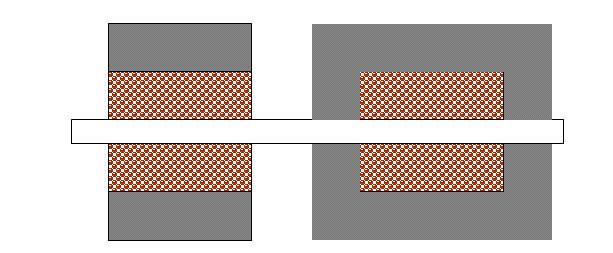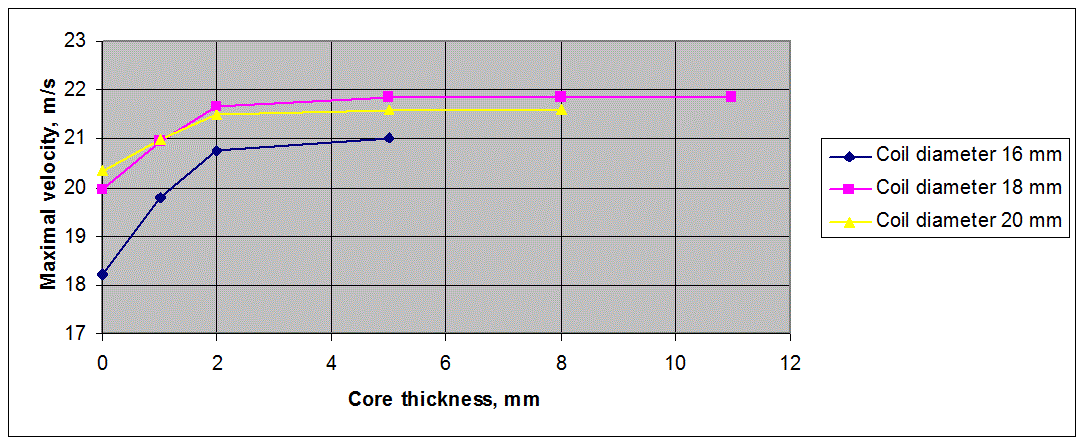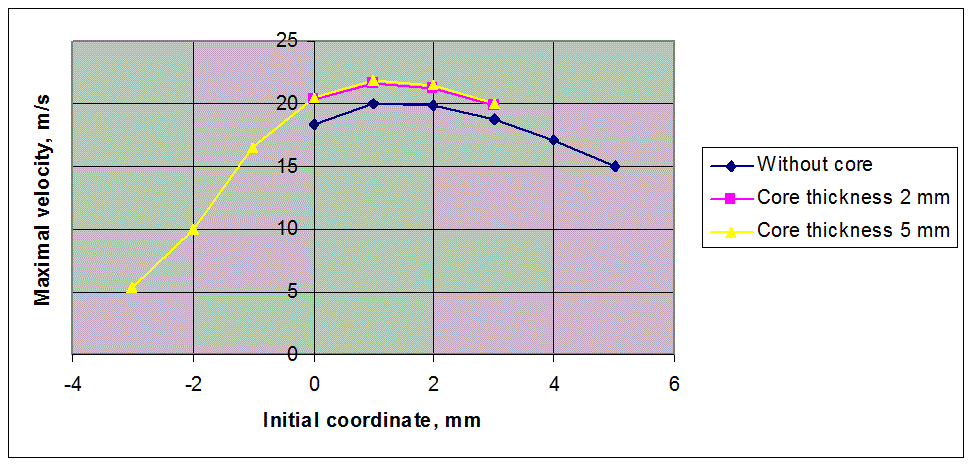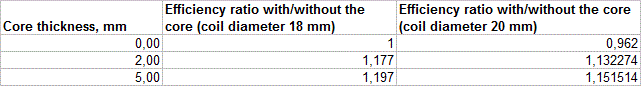Influence of the magnetic core on efficiency of the gauss gun

The following calculations were executed when I was projecting a multistage ball-firing coilgun, and trying to utilize a magnetic core in its construction. Slightly modified script http://coilgun.ucoz.ru/FEMM_calc/Stator/gauss_ver_2_5.lua for SCR-driven coilgun modelling was used. The tubular core (fig. 1) is suggested here with a coil inside it. Such form is chosen because:

- it is easy to make (for example by winding steel tape arong the coil)

- ts suitability for multistage systems where the coils must be placed end-to-end (so "traditional" core with its "cheeks" adjoining to the coils is not appropriate)Fig. 1. Cross-section of the core modelled in initial (right) and adapted script (left).

Other parameters of the system were:

- capacity of 400 mkF;

- initial voiltage 300 V;

- ESR 60 mOhm (according to the measurements of K50-17 caps);

- parasitic circuit resistance 50 mOhms (open SCR plus wire resistance);

- inside coil diameter 10 mm;

- projectile diameter 8 mm (steel ball);

- initial speed 0 m/s (i.e. the first stage was modelled).

The procedure of modelling lookes like follows: first, the length of the coil and wire gauge giving maximum efficiency (i.e. velocity of the projectile) are determined without any core. Then, more precise calculation is proceeded in a range of outside coil diameters and initial (starting) position of the projectile. At last, the calculation repeats accounting the core of variable thickness (suggesting it is solid iron closely adjoining to the winding, which of course is some sort of idealization).

Here is what we have as a result of this process:Fig. 2. Maximum speed vs core thickness around the coils of different diameters.Fig. 3 Maximum speed vs initial coordinate.

As we can see, the core increases max. efficiency, but only slightly. It is unreasonable to take the core thicker than 5 mm - obvioulsy it corresponds to its  full saturation. The core's influence on the optimal initial position is absent.

According to the evaluation, best case is outside diameter 20 mm for the "bold" coil and 18 mm with the core. The table below gives comparison of efficiencies (proportional to the squared velocities) for those two thicknesses with and without the core.It means that if we take a coil giving the best efficiency and place it into a core, we can only have 15% increase of efficiency.

It should be noted that these evaluations are true for relatively low-energy system and don't account for eddy-current loses. In reality, there is more energy utilized in each stage of multisatge coilguns, so the advantages of the core would be even less (because it will be saturated faster).

As a result, I decided not to use the magnetic core in this (and all following) project.

'